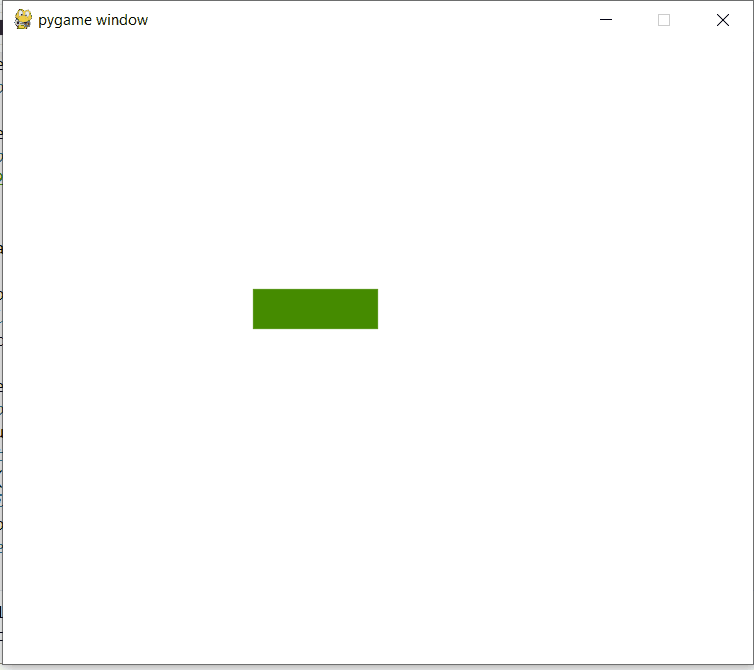# How to create a text input box with Pygame?

• Difficulty Level : Basic
• Last Updated : 26 Mar, 2021

In this article, we will discuss how to create a text input box using PyGame.

### Installation

Before initializing pygame library we need to install it. This library can be installed into the system by using pip tool that is provided by Python for its library installation. Pygame can be installed by writing these lines into the terminal.

We can install Pygame using command :

`pip install pygame `

Pygame can be used to create a text input box which will be explained step by step further in this article.

#### Approach

1. Use pygame.init() which will initialize all imported modules.
2. Set screen size.
3. Set font of the text which user will type.
4. Create a condition according to user key.
5. Also, declare two variable which will contain color name which will be further used for input color.
6. Also, store input in a variable to display on screen.
7. Now draw rectangle and pass argument which should be on screen.
8. Also, set the size of screen to be rendered.
9. Use clock.tick() which means that for every second at most given frames should be passed.

## Python3

 `# import sys module``import` `pygame``import` `sys`` ` ` ` `# pygame.init() will initialize all``# imported module``pygame.init()`` ` `clock ``=` `pygame.time.Clock()`` ` `# it will display on screen``screen ``=` `pygame.display.set_mode([``600``, ``500``])`` ` `# basic font for user typed``base_font ``=` `pygame.font.Font(``None``, ``32``)``user_text ``=` `''`` ` `# create rectangle``input_rect ``=` `pygame.Rect(``200``, ``200``, ``140``, ``32``)`` ` `# color_active stores color(lightskyblue3) which``# gets active when input box is clicked by user``color_active ``=` `pygame.Color(``'lightskyblue3'``)`` ` `# color_passive store color(chartreuse4) which is``# color of input box.``color_passive ``=` `pygame.Color(``'chartreuse4'``)``color ``=` `color_passive`` ` `active ``=` `False`` ` `while` `True``:``    ``for` `event ``in` `pygame.event.get():`` ` `      ``# if user types QUIT then the screen will close``        ``if` `event.``type` `=``=` `pygame.QUIT:``            ``pygame.quit()``            ``sys.exit()`` ` `        ``if` `event.``type` `=``=` `pygame.MOUSEBUTTONDOWN:``            ``if` `input_rect.collidepoint(event.pos):``                ``active ``=` `True``            ``else``:``                ``active ``=` `False`` ` `        ``if` `event.``type` `=``=` `pygame.KEYDOWN:`` ` `            ``# Check for backspace``            ``if` `event.key ``=``=` `pygame.K_BACKSPACE:`` ` `                ``# get text input from 0 to -1 i.e. end.``                ``user_text ``=` `user_text[:``-``1``]`` ` `            ``# Unicode standard is used for string``            ``# formation``            ``else``:``                ``user_text ``+``=` `event.``unicode``     ` `    ``# it will set background color of screen``    ``screen.fill((``255``, ``255``, ``255``))`` ` `    ``if` `active:``        ``color ``=` `color_active``    ``else``:``        ``color ``=` `color_passive``         ` `    ``# draw rectangle and argument passed which should``    ``# be on screen``    ``pygame.draw.rect(screen, color, input_rect)`` ` `    ``text_surface ``=` `base_font.render(user_text, ``True``, (``255``, ``255``, ``255``))``     ` `    ``# render at position stated in arguments``    ``screen.blit(text_surface, (input_rect.x``+``5``, input_rect.y``+``5``))``     ` `    ``# set width of textfield so that text cannot get``    ``# outside of user's text input``    ``input_rect.w ``=` `max``(``100``, text_surface.get_width()``+``10``)``     ` `    ``# display.flip() will update only a portion of the``    ``# screen to updated, not full area``    ``pygame.display.flip()``     ` `    ``# clock.tick(60) means that for every second at most``    ``# 60 frames should be passed.``    ``clock.tick(``60``)`

Output:My Personal Notes arrow_drop_up